# Real Numbers - Olympiad Level MCQ, Class 10 Mathematics

## 40 Questions MCQ Test Olympiad Preparation for Class 10 | Real Numbers - Olympiad Level MCQ, Class 10 Mathematics

Description
Attempt Real Numbers - Olympiad Level MCQ, Class 10 Mathematics | 40 questions in 40 minutes | Mock test for Class 10 preparation | Free important questions MCQ to study Olympiad Preparation for Class 10 for Class 10 Exam | Download free PDF with solutions
QUESTION: 1

### Q. The greatest number which on dividing 1657 and 2037 leaves remainders 6 and 5 respectively, is:

Solution:

Required number = H.C.F. of (1657 - 6) and (2037 - 5)

= H.C.F. of 1651 and 2032 = 127.

QUESTION: 2

Solution:
QUESTION: 3

### The largest possible number with which when 38, 66 and 80 are divided,leaves the remainder same is –

Solution:

Let the common remainder be  10

From the given numbers we now subtract the common remainder

i.e 38 − 10 = 28,66 −10 = 56, 80 −10 = 70

Now  we will find the HCF of 28, 56, 70

28 = 2 x 7 x 2

56 = 2 x 2 x 2 x 7

70 = 2 x 5 x 7

So HCF of 28, 56 and 70 = 2 x 7

So the required number is 14

QUESTION: 4

What is the least possible number which when divided by 24, 32 or 42 in each case it leaves the remainder 5?

Solution:

LCM of 24 , 32 & 42 = 672

The least possible number for your given case = 672 +5 = 677

QUESTION: 5

What is the least possible number which when divided by 24, 32 or 42 in each case leaves remainder 5. How many such numbers are possible between 666 and 8888?

Solution:
QUESTION: 6

The least number which when divided by 5, 6 , 7 and 8 leaves a remainder 3, but when divided by 9 leaves no remainder, is:

Solution:

L.C.M. of 5, 6, 7, 8 = 840.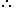Required number is of the form 840k + 3

Least value of k for which (840k + 3) is divisible by 9 is k = 2.Required number = (840 x 2 + 3) = 1683.

QUESTION: 7

What is the least possible number which when divided by 18, 35 or 42 leaves 2, 19, 26 as the remainders
respectively?

Solution:

N= 18a+2= 35b+19=42c+26

=> N+16= 18a=35b=42c

=> N+16 is divisible by LCM (18,35,42)

=> N+16 is divisible by 630 (=3*3*2*5*7)

=> N= 630k-16

=> Least possible number= 630*1-16= 614

QUESTION: 8

What is the least possible number which when divided by 2, 3, 4, 5, 6 leaves the remainders 1, 2, 3, 4, 5 respectively?

Solution:

Here,
2 - 1 = 1
3 - 2 = 1
4 - 3 = 1
5 - 4 = 1
6 - 5 = 1
That is, if we subtract 1 from the number divisible by 2, 3, 4, 5, 6, we get the required number.

The smallest number divisible by 2, 3, 4, 5, 6 will be the LCM of 2, 3, 4, 5, 6.

LCM of 2,3, 4, 5, 6 is 60
So, the required number which leaves a remainder of 1,2,3,4,5= L.C.M of (2,3,4,5,6)-1=59

QUESTION: 9

The least number which when divided by 2, 3, 4, 5 and 6 leaves the remainder 1 in each case. If the same number is divided by 7 it leaves no remainder. The number is

Solution:

The least common multiple of 2, 3, 4, 5, 6 is their LCM=60.
So, the answer is the least number such that it is a multiple of 7 and a multiple of 60 leaving remainder 1.

QUESTION: 10

How many numbers lie between 11 and 1111 which when divided by 9 leaves a remainder of 6 and when divided
by 21 leaves a remainder of 12?

Solution:

Number lies between 11 and 1111 When divide by 9 a remainder of 6 and when divided by 21 leave a remainder of 12 = 33, 96, 159 (all separated by 63)

a =33  d=63
1111=a+(n-1)63

1111=33+(n-1)63

1078=(n-1)63

1078/63=n-1

n=(1078+63)/63

n= 1141/63

n = 18.11

then total number=18

QUESTION: 11

The largest number which divides 70 and 125, leaving remainders 5 and 8 respectively, is

Solution:

Since, 5 and 8 are the remainders of 70 and 125, respectively. Thus, after subtracting these remainders from the numbers, we have the numbers 65 = (70-5),
117 = (125 – 8), which is divisible by the required number.
Now, required number = HCF of 65,117                 [for the largest number]
For this, 117 = 65 x 1 + 52 [∵ dividend = divisor x quotient + remainder]
⇒ 65 = 52 x 1 + 13
⇒ 52 = 13 x 4 + 0
∴ HCF = 13
Hence, 13 is the largest number which divides 70 and 125, leaving remainders 5 and 8.

QUESTION: 12

If x|y, where x > 0, y > 0 (x, y ε z) then –

Solution:
QUESTION: 13

If a|b, then gcd of a and b is –

Solution:

Let a|b and the GCD(a,b) = m, then b=aq for some integer q and the GCD(a,b) can be expressed as a linear combination with some integer x and y,

ax + by = m .

Substituting in b we get,

ax + (aq)y = m

a(x + qy) = m

But x + qy = GCD(1,q) =1. Thus,

a(1) = m

Hence a = GCD(a,b), which is what we needed to show.

QUESTION: 14

A number 10x + y is multiplied by another number 10a + b and the result comes as 100p + 10q +r, where r = 2y, q = 2(x + y) and p = 2x; x, y < 5, q ≠ 0. The value of 10a + b may be:

Solution:

According to problem
(10x+y)(10a+b)=100p + 10q + r
By substituting the values
r=2y
q=(2x+y)
p=2x
(10x+y)(10a+b)=100*2x+10*2(x+y)+2y
(10x+y)(10a+b)=220x+22y
10a+b=22

QUESTION: 15

If x, y ε R and |x| + |y|= 0, then –

Solution:
QUESTION: 16

If a, b, c ε R and a2 + b2 + c2 = ab + bc + ca, then –

Solution:

Consider,

a2 + b2 + c2 – ab – bc – ca = 0

Multiply both sides with 2, we get
2( a2 + b2 + c2 – ab – bc – ca) = 0
⇒ 2a+ 2b2 + 2c2 – 2ab – 2bc – 2ca = 0

⇒ a2 + a+ b2 + b+ c2 + c2– 2ab – 2bc – 2ca = 0
⇒ (a2 – 2ab + b2) + (b2 – 2bc + c2) + (c2 – 2ca + a2) = 0
⇒ (a – b)2 + (b – c)2 + (c – a)2 = 0
Since the sum of squares is zero, it means each term should be zero.
⇒ (a – b)2 = 0,  (b – c)2 = 0, (c – a)2 = 0
⇒ a – b = 0,  b – c = 0,  c – a = 0
⇒ a = b,  b = c, c = a
⇒ a = b = c

QUESTION: 17

If x, y ε R and x < y ⇒ x2 > y2 then –

Solution:
QUESTION: 18

If x, y ε R and x > y ⇒ |x| > |y|, then–

Solution:
QUESTION: 19

If x, y ε R and x > y ⇒ |x| < |y|, then–

Solution:
QUESTION: 20

π and e are –

Solution:

π (Pi) and e are famous irrational numbers

π = 3.1415926535897932384626433832795... (and more)

e = 2.7182818284590452353602874713527 (and more ...)

We cannot write down a simple fraction that equals Pi.

The popular approximation of  22/7 = 3.1428571428571... is close but not accurate.

The first few terms of e add up to: 1 + 1 + 1/2 + 1/6 + 1/24 + 1/120 = 2.71666...

QUESTION: 21

If a, b ε R and a < b, then

Solution:
QUESTION: 22

If x is a non-zero rational number and xy is irrational, then y must be –

Solution:

The product of a rational and irrational number is irrational.

2 × √5 = 2√5 is an irrational number.

Product of a rational and irrational number always irrational if rational number is not zero.

QUESTION: 23

The arithmetical fraction that exceeds it's square by the greatest quantity is –

Solution:
QUESTION: 24

If x and y are rational numbers such that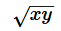is irrational, then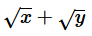is

Solution:

Take an example of 2 and 3.
When you multiply,
√2×√3=√6(Irrational).
√2+√3=Irrational.

QUESTION: 25

If x and y are positive real numbers, then –

Solution:
QUESTION: 26

If two positive integers a and b are written as a = x3y2 and b = xy3; x, y are prime numbers, then HCF (a, b) is

Solution:

Given that, a = x3y2 = x x x x x x y x y
and b = xy3 = x x y x y x y
∴ HCF of a and b = HCF (x3y2, xy3) = x x y x y = xy2
[Since, HCF is the porduct of the smallest power of each common prime facter involved in the numbers]

QUESTION: 27

If x ε R, then |x|=

Solution:
QUESTION: 28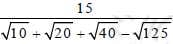is equal to –

Solution:

Explanation : 15/(10)1/2 + (20)1/2+ (40)1/2- (125)1/2

(3*5)/[(2)1/2(5)1/2 + 2(5)1/2 + 2(2)1/2(5)1/2 - 5(5)1/2]

= (3*5)/[3(2)1/2(5)1/2 - 3(5)1/2]

= (3*5)/3(5)1/2[(2)1/2 - 1]

= (5)1/2/[(2)1/2 - 1]

Rationalise it, we get

= (5)1/2/[(2)1/2 - 1] * [(2)1/2 + 1]/[(2)1/2 + 1]

= (5)1/2[(2)1/2 + 1]/(2 - 1)

= (5)1/2/[(2)1/2 + 1]

QUESTION: 29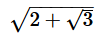+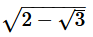is equal to –

Solution:
QUESTION: 30

The expression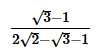is equal to –

Solution:
QUESTION: 31

If x, y, z are real numbers such that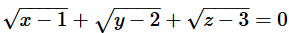then the values of x, y, z are respectively

Solution:
QUESTION: 32

if a, b, c ε R and a > b ⇒ ac < bc, then –

Solution:
QUESTION: 33

If a, b, c ε R and ac = bc ⇒ a = b, then –

Solution:
QUESTION: 34

Between any two distinct rational numbers –

Solution:

Infinite number of rational numbers exist between any two distinct rational numbers. We know that a rational number is a number which can be written in the form of p/q​ where p and q are integers and q not equal to 0.

QUESTION: 35

The total number of divisors of 10500 except 1 and itself is –

Solution:

10,500 = 2^2 x 3^1 x 5^3 x 7^1

d(n) = (a + 1)(b + 1)(c + 1)(d + 1)

d(10500) = (2 + 1)(1 + 1)(3 + 1)(1 + 1)

d(10500) = (3)(2)(4)(2)

d(10500) = 48

Now 1 and 10500 should not be counted

so number of divisors = 48 - 2 = 46

QUESTION: 36

The sum of the factors of 19600 is –

Solution:

Properties of the number 19600

Factorization

-

2 * 2 * 2 * 2 * 5 * 5 * 7 * 7

Divisors- 1, 2, 4, 5, 7, 8, 10, 14, 16, 20, 25, 28, 35, 40, 49, 50, 56, 70, 80, 98, 100, 112, 140, 175, 196, 200, 245, 280, 350, 392, 400, 490, 560, 700, 784, 980, 1225, 1400, 1960, 2450, 2800, 3920, 4900, 9800, 19600

Count of divisors- 45

Sum of divisors - 54777

QUESTION: 37

The product of divisors of 7056 is –

Solution:
QUESTION: 38

The number of odd factors (or divisors) of 24 is –

Solution:

24 can be divided by two odd numbers which are 1 and 3 only.

QUESTION: 39

The number of even factors (or divisors) of 24 is –

Solution:

Factors of 24 are 2, 3 ,4 6 8 12 and 24 itself
therefore , no of even factors are 6

QUESTION: 40

In how many ways can 576 be expressed as a product of two distinct factors?

Solution:

576 = 26 x 32
∴ Total number of factors = (6 + 1) (2 + 1) = 21
So the number of ways of expressing 576 as a product of two distinct factors =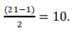Use Code STAYHOME200 and get INR 200 additional OFF Use Coupon Code